#### New products

» Do not allow new products at this time.

#### Recent posts

• #####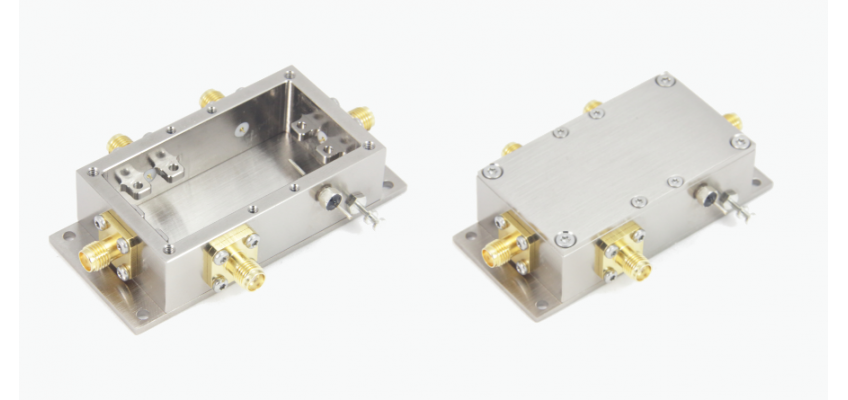RF Enclosure Mini-Extended-FX: a...
Sep 15, 2021 4801
• #####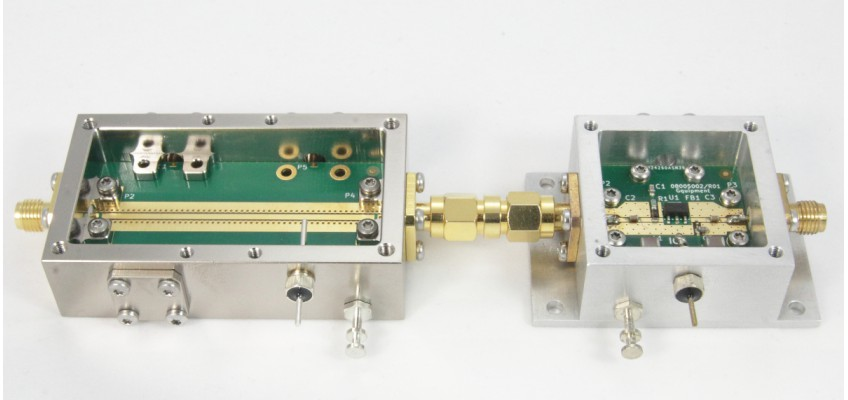Aluminum RF and mmWave Enclosures
Aug 22, 2021 5806
• #####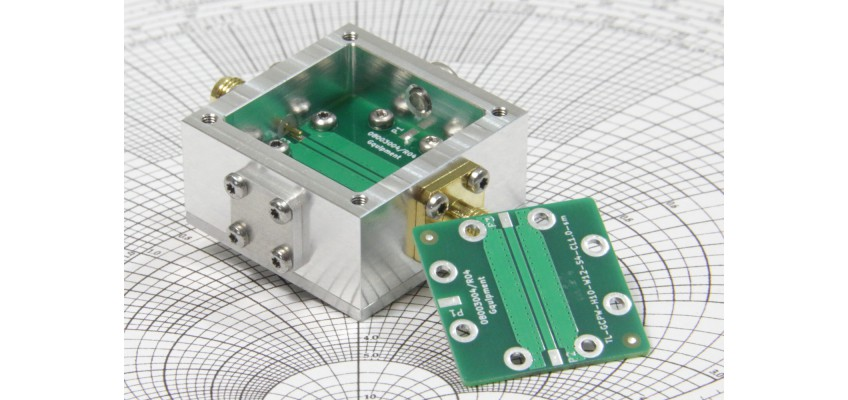SMA connector performance in the...
Feb 6, 2021 7341
• #####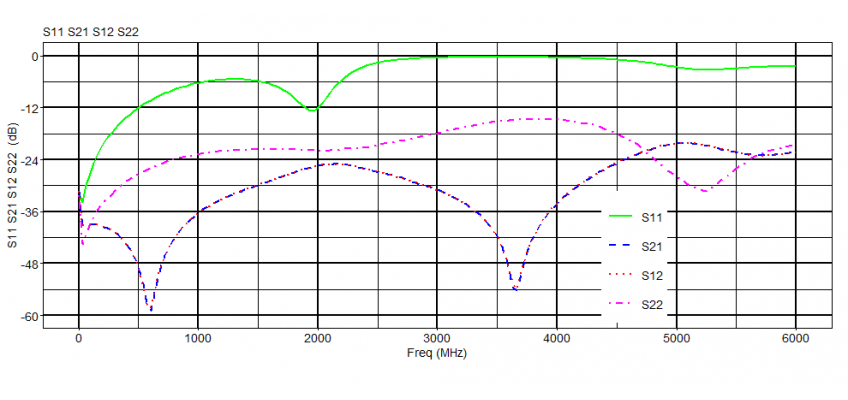Online S-parameter viewer
Nov 15, 2020 10676
• #####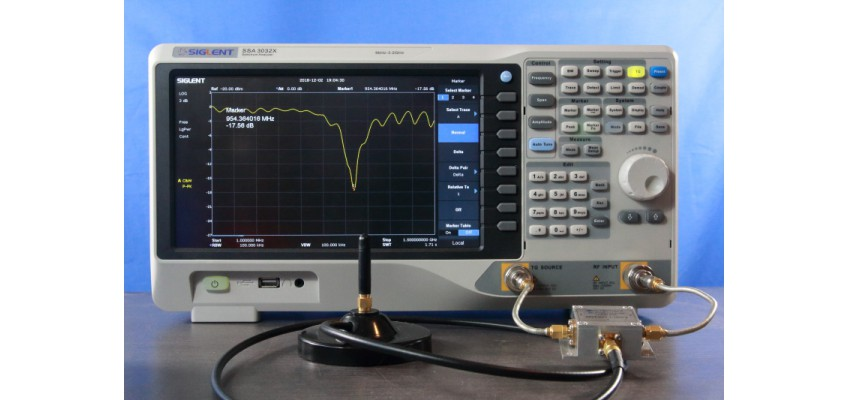Everything You Should Know About...
May 13, 2019 31144

# Modeling Devices with S-parameters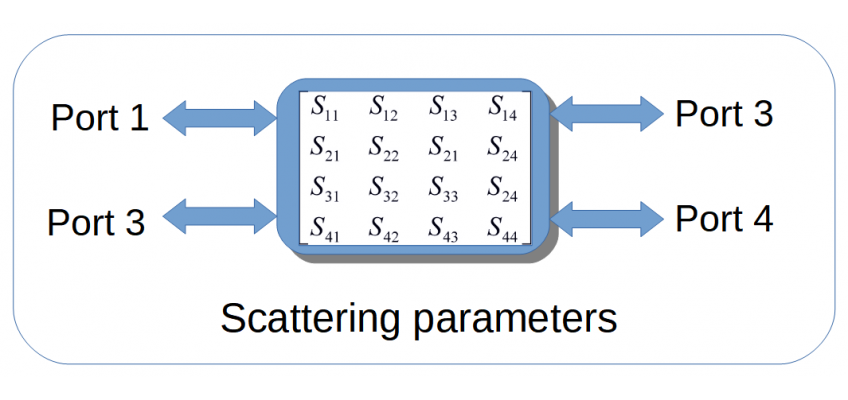Scattering parameters or S-parameters for short, are the standard way to describe the behaviour of all kind of RF devices. Both passive and active. So, what are these parameters and why are they so popular? In order to fully grasp the full potential of S -parameters, it is useful to lay down some basic concepts first.

## 1. Concepts

The basic idea behind S-parameters is to look at a device (or more generally, a network) as a black box. Where only the input and output ports can be interacted with. Each port has two terminals. It’s the voltage difference between the terminals that matters here. Current that travels into one terminal must come out of the other. Each port has an impedance (‘Z’) associated with it. Usually this impedance is 50 Ohms.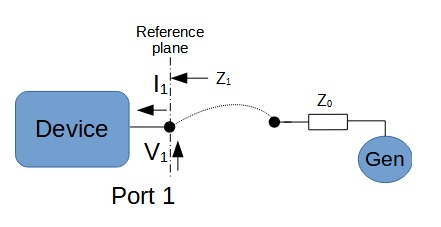Figure 1. Example of a one port device.

The next important concept is that we are always talking about (power) waves that travel through these ports. Power waves can both enter or exit a port. Because we are talking about traveling waves, transmission line theory must be applied.

If we connect a transmission line (in practice: a coaxial cable) to a port and the impedance of the port and the transmission line are exactly matched, all power will be transferred to the port. However, if there is a mismatch, then there will be some power reflected back into the line.

The S-parameter universe is defined around the power waves that are incident to a port (‘a’) and the reflected wave ('b'), instead of talking about voltages and currents.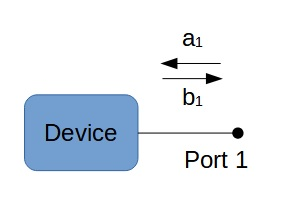Figure 2. Incident (a1) and reflected wave (b1).

In this section we’ve restricted ourselves to a one-port device so far. Of course, there are devices with more then one port too! In general, a device can have ‘N’ ports. In the following figure an example is given of a two- and three- port device.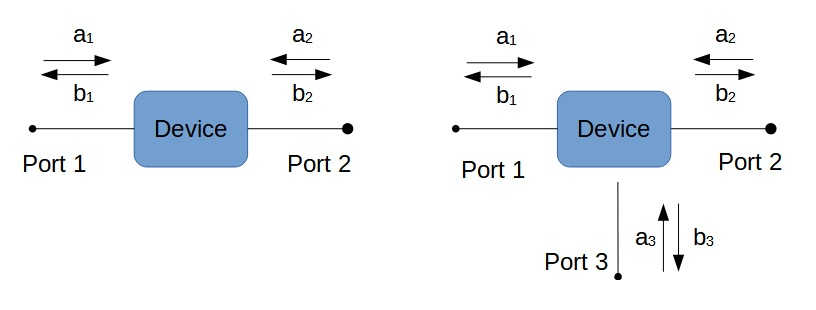Figure 3. Example of two and three port devices.

A good example of a one-port device is a 50 Ohm terminator or an antenna. An attenuator or a filter are exemplary for two-port devices. While a power splitter can be modeled as a three-port device.

### 1.1 S-parameters and Their Physical Representation

Now we are ready to explain what a S-parameter is and what it means. An S-parameter of a device is a ratio between the (power) waves at and between its ports. As there are both incident- and reflected- waves and one or more ports, it follows that a device in general will have many S-parameters to model its behaviour.

We will break this down for a one-, two- and three- port device.

With a one-port device there is no ratio of power waves between its port as there is only one port. That leaves us with the only ratio that does exist - the ratio between the reflected power versus the incident power wave. That is the ratio b1 / a1, and is denoted with S11. This is called the reflection coefficient or return loss. Which is also the physical manifestation of S11.

Next is the two-port devices. Our first observation is that for each individual port there is an S11 and an S22 reflections coefficient. But now there are also power wave ratio's possible between ports 1 and 2. The first one is the relation S21 which is defined as the power wave ratio of b2/a1 (while keeping a2 at zero so that b2 is merely accounted for by a1). The name of this parameter (S21) is insertion loss. Again, this is a good way to describe its physical expression when the two-port device is a cable or some sort of attenuator. If the device is an amplifier, gain is the preferred name of S21.

The second port-to-port relation is S12 , which equals b1/a2. This is the ratio of the incident wave at port 2 and the power wave leaving port 1 (while keeping a1 at zero). The name of S12 is reverse isolation. It can be shown that in a passive device S21 and S12 are always equal. Which intuitively makes sense, since the insertion loss and the reverse isolation of a cable will clearly be the same. However, if we look at an amplifier, gain and reverse isolation will most likely be different and here the meaning of reverse isolation is self- explanatory.

In the next figure you will find a summary of the three types of S-parameters we looked at so far.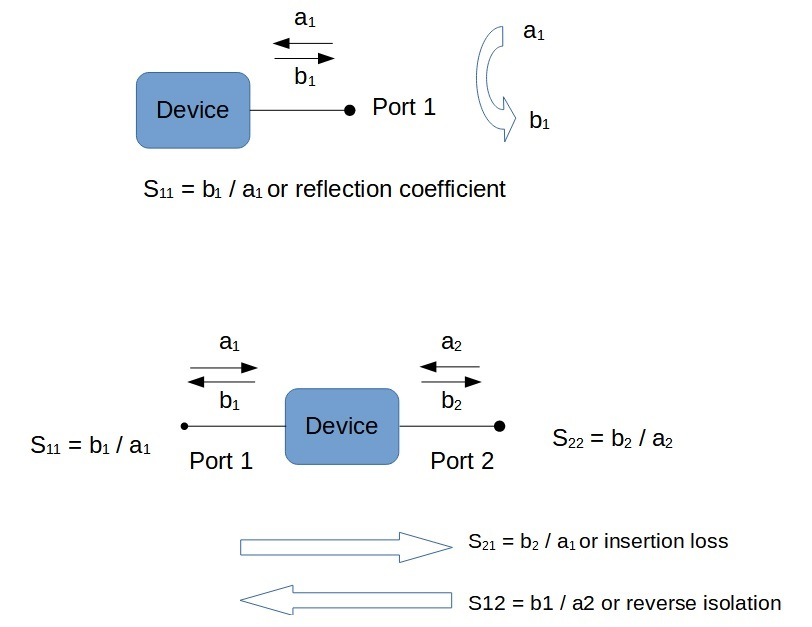Figure 4. Summary of reflection coefficient, insertion loss or gain and reverse isolation.

In this section the emphasis is on the physical meaning of S-parameters. Which depends on the context the device is used in. The S-parameters are always just a ratio of two power waves. We will finish this paragraph with another example of the meaning of S-parameters by looking at a three port device: the power combiner (see figure below).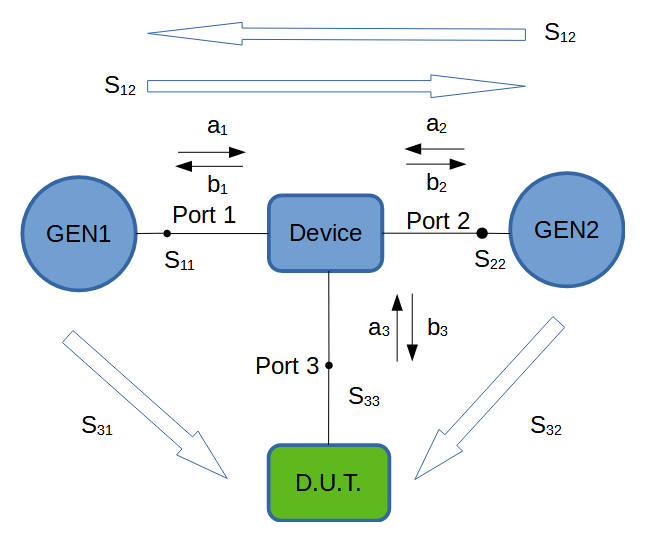Figure 5. S-parameters of a power combiner

The function of a power combiner is to combine the power waves from the generators GEN1 and GEN2. This is a quite common test setup for performing Inter Modulation Distortion (IMD) measurements. The D.U.T. could be some sort of amplifier. In this context it is important that both signals are equally added and fed to the D.U.T. So S31 must equal S32. The second concern in this type of measurement setup is that GEN1 does not influence GEN2 and vice versa. They are typically operated at frequencies that are very close together. So S12 and S21 should be as small as possible. Note that they will also be equal as a combiner is a passive device. In this situation, S21 and S12 are called the isolation parameters of the device. For these types of measurements, an splitter with an isolation of 20dB or higher is recommended.

### 1.2 Frequency-dependent

Devices described by S-parameters always show some sort of frequency-dependent behaviour. This is by design. For example the device should pass low frequencies only. Or, alternatively, they are designed to do something which should be as frequency-independent as possible. As is the case with an attenuator or amplifier. In either case, we are always interested in the S-parameters for a frequency range of interest. S-parameters are therefore presented in tabular or graphical form.

### 1.3 Linear Systems Only

Please note that S-parameters only apply to linear systems. When a set of S-parameters is given for a BJT transistor, it will be valid for small signal analyses only.

### 1.4 Amplitude and Phase

When modeling the properties of a device (like insertion loss or gain), we are generally interested in both the magnitude and phase information of the transfer function. Therefore, S-parameters are complex numbers that model both these properties. The same goes for other quantities like Z, V, I or the reflection coefficient Γ used in this article.

## 2. Definitions

In this section we will take a more formal and general approach to the description of devices by means of S-parameters. The purpose is not to give an elaborate treatment of the subject-matter, but to point out some important issues that (hopefully) will assist you when working with S-parameters.

### 2.1 Notation and Definitions

The relations between the power waves from figure 4 (ax and bx) can be written down in the following equations:

b1 = S11 . a1 + S12 . a2

b2 = S21 . a1 + S22 . a2

These type of linear equations lend themselves well to writing down in vector notation. This will prove quite handy when we are working with devices with more than two ports.

So, b = S . a, with the vectors a and b containing the successive power waves 'ax' and 'bx'. S is the matrix containing all the S-parameters.

For the set of equations above, S looks like: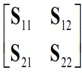The formal definitions of the S-parameters are: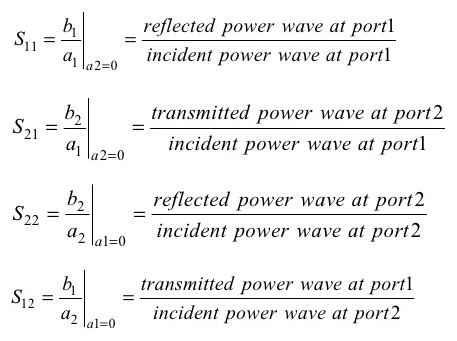### 2.3 What About that Power Wave?

In the definitions above and in Chapter 1, we already talked about power waves 'a' and 'b'. However, this was done without a proper introduction. We will now take a closer look at this phenomenon, but without the mathematical treatment.

We start the discussion by introducing the definitions of both 'a' and 'b' for a port: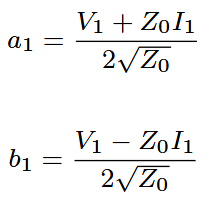V and I are the complex voltage and current flowing into the port 1 with impedance Z0. It can be shown that |a1|2 and |b1|2 correspond to the power of the incedent wave and the power of the reflected wave. This derivation starts with the notion that power is transferred between a generator and the port using V, I and Z as parameters. Then a linear transformation is carried out using the above definition of a1 and b1. This transformation just gives us another projection to look at the power transfer. Not in terms of V and I but in a1 and b1. It can then be shown that a1 and b1 have a clear physical meaning, as the incident and reflected power. For those looking at the exact derivation, the article of K. Kurokawa is recommended (1965, "Power Waves and the Scattering Matrix").

As a1 and b1 stand for the traveling waves at the port, they correspond with the forward and backward traveling (reflected) waves V+ and V- along a transmission line. The only difference is that a1 and b1 are normalized at the square root of Z (1 / Z). It is therefore not uncommon to find S-parameters calculated by using the traveling wave parameters V+ and V-.

### 2.3.1 Converting S-parameters to dB

We know that S-parameters can be calculated from the ratios of the traveling waves amplitudes V+ and V-. The square of these values correspond with the wave power |ax|2. So when we want to express an S-parameter value in dB, we have to use the formula 20Log(S).

Converting S-parameters to dB is often very convenient. It helps us to cope with a broad range of values. Which is the primary goal of the dB unit anyway. Some examples are (referring to figure 4):

Gain : 20Log (S21) dB

Input return loss : -20 Log (S11) dB

## 3. Relation between Sxx and Impedance Mismatch

It is important to realize that S11 is equivalent to the reflection coefficient Γ as defined in transmission theory. Both define the amount of reflected power. We also know that the amount of reflected energy at the end of a transmission line is defined by the impedance mismatch between Z0 and ZL (see figure 6). Therefore, if we know the reflection coefficient S11 and Z0, the impedance ZL can be calculated.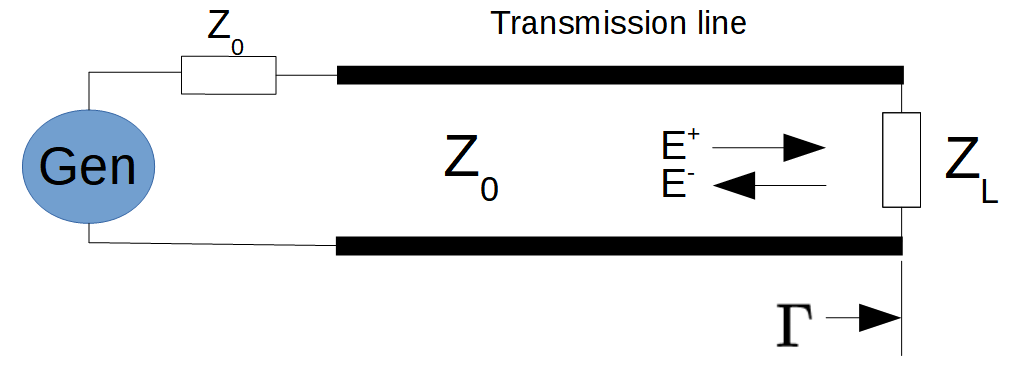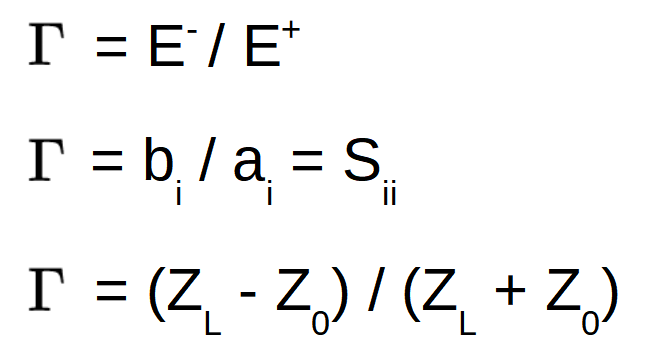Figure 6. Reflection coefficient and relation with Zo and ZL

So, port impedance can be calculated by measuring the reflection coefficient (or return loss).

${displaystyle Gamma }$

## 4. Measuring S-parameters

One of the advantages of using S-parameters is that they are relative easily measured due to the 50 Ohms reference impedance of the test ports. Compare this with the Y- and Z-parameter measurements. These are both difficult to measure and demanding for the D.U.T. Largely due to the test procedure which uses either open or shorted ports.

The measurement of S-parameters is typically done with a Vector Network Analyzer (VNA) at the press of a button. Regular calibration of the measurement setup is an important step in this procedure, which often is taken care of by the VNA as well. The VNA will store the set S-parameters in a file that can be used as the device's S-parameters model. These files can be imported into network simulation software to analyze RF networks.

It is also possible to measure S-parameters with a Spectrum Analyser (SA). However the results will not be complete as an SA cannot measure phase. Due to this lack of phase information it is also impossible to implement the error-correction steps that VNAs use to get to their precise measurements. This is the reason why the use of an SA for measuring S-parameters can be very challenging at times.

## 5. Using S-Parameters

As should be obvious by now, S-parameters are used to model all kinds of RF devices. These models are stored in S-parameter files by using the widely accepted Touchstone standard. Most RF simulators can import and use them to model the device in some RF network. There are a lot of on-line Touchstone viewers too.

Of course, a device can also evaluated by studying its S-parameter graphs that show properties like insertion loss or gain, return loss, and isolation. To name the most common parameters.

Note that S-parameters are also used to model RF transistors. Although a transistor has three terminals, in all basic amplifier configuration there is always one terminal shared. Which leaves us with a two-port configuration. So don't expect three-port S-parameter models with transistors.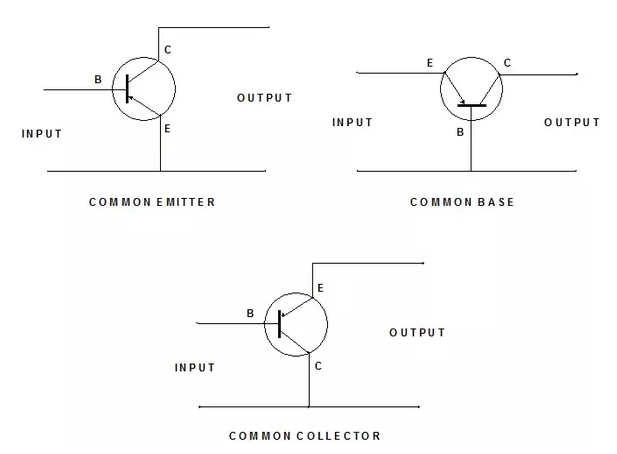Figure 7 Two port model of basic transistor configurations

## 6. Conclusion

S-parameters are an invaluable way to model RF devices. They are relatively easy to measure, can be used in RF circuit simulators and are a good way to evaluate RF devices because of the clear physical meaning of the S-parameters themselves.

It comes as no surprise that S-parameters are used extensively in the RF industry.